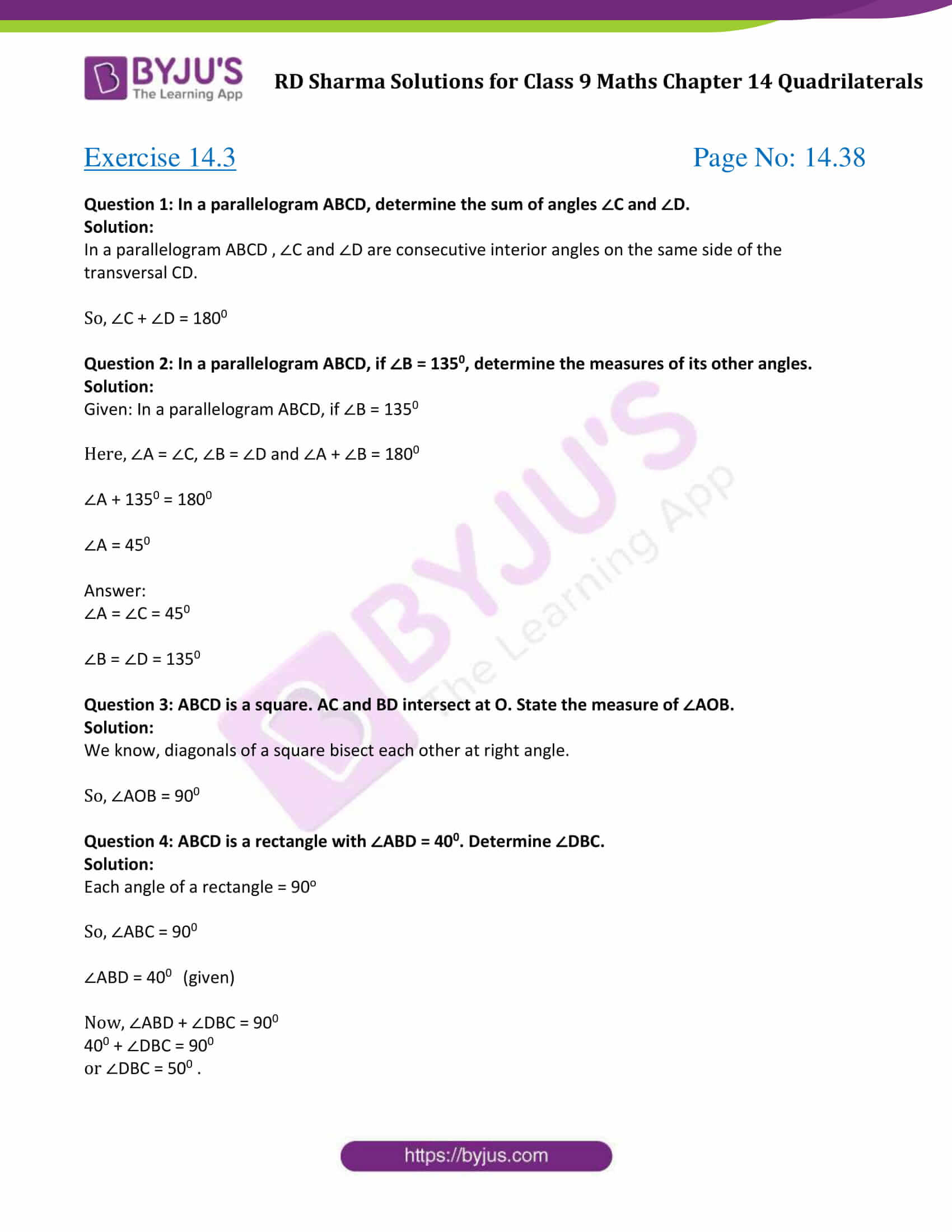# RD Sharma Solutions for Class 9 Maths Chapter 14 Quadrilaterals Exercise 14.3

RD Sharma class 9 Mathematics chapter 14 exercise 14.3 solutions for Quadrilaterals are provided here. In this exercise, students will learn about conditions for a quadrilaterals to be a parallelogram and some important theorems results. Students can download all the exercise 14.3 of  RD Sharma Class 9 Solutions in the form of pdf by clicking on the given link below.### Access Answers to Maths RD Sharma Solutions for Class 9 Chapter 14 Quadrilaterals Exercise 14.3 Page number 14.38

Question 1: In a parallelogram ABCD, determine the sum of angles ∠C and ∠D.

Solution:

In a parallelogram ABCD , ∠C and ∠D are consecutive interior angles on the same side of the transversal CD.

So, ∠C + ∠D = 1800

Question 2: In a parallelogram ABCD, if ∠B = 1350, determine the measures of its other angles.

Solution:

Given: In a parallelogram ABCD, if ∠B = 1350

Here, ∠A = ∠C, ∠B = ∠D and ∠A + ∠B = 1800

∠A + 1350 = 1800

∠A = 450

∠A = ∠C = 450

∠B = ∠D = 1350

Question 3: ABCD is a square. AC and BD intersect at O. State the measure of ∠AOB.

Solution:

We know, diagonals of a square bisect each other at right angle.

So, ∠AOB = 900

Question 4: ABCD is a rectangle with ∠ABD = 400. Determine ∠DBC.

Solution:

Each angle of a rectangle = 90o

So, ∠ABC = 900

∠ABD = 400 (given)

Now, ∠ABD + ∠DBC = 900

400 + ∠DBC = 900

or ∠DBC = 500 .

## RD Sharma Solutions for Class 9 Maths Chapter 14 Quadrilaterals Exercise 14.3

RD Sharma Solutions Class 9 Maths Chapter 14 Quadrilaterals Exercise 14.3 is based on the following topics:

1. Conditions for a Quadrilaterals to be a Parallelogram

2. Important theorems statements:

• A quadrilateral is a parallelogram if its opposite sides are equal.
• A quadrilateral is a parallelogram if its opposite angles are equal.
• If the diagonals of a quadrilateral bisect each other, then the quadrilateral is a parallelogram.
• A quadrilateral is a parallelogram if its one pair of opposite sides are equal and parallel.
• Properties of Rectangle, Rhombus and a Square
• Theorems on Rectangle, Rhombus and a Square